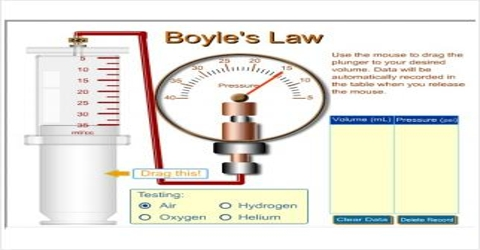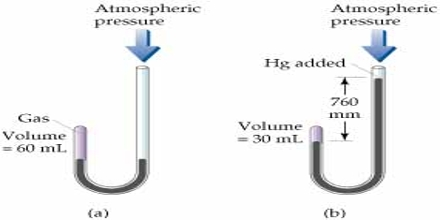Chemistry

# Boyle’s Law: Explanation in terms of Gaseous StateBoyle’s Law

The effect of pressure on the volume of a gas was extensively studied by Robert Boyle in 1662. He enclosed some gas at the short end of a J-shaped tube and poured mercury at the other end. He found that the volume of the gas decreased (Figure). Each addition of mercury meant increase of pressure and this resulted in decrease of volume of the gas. The volume of a given mass of gas is 100 ml at atmospheric pressure. The volume is halved when the pressure is doubled by adding Hg. The volume is decreased to 33 ml by tripling the pressure to 1520 mm Hg. From such experiments he formulated a law of gases, known as Boyle’s law.According to this law,

At constant temperature a fixed mass of gas occupies a volume which is inversely proportional to the pressure exerted on it.

If the pressure is doubled the volume becomes one-half of the original. Expressed mathematically the law states that;

V∞ (1/P) [n and T constant]

or, P.V = Constant [n and T constant]

Here n is the number of moles of gases and T is Temperature.

It says that if a given mass of gas occupies a volume V1 at a pressure of P1 and a volume V2 at a pressure of P2 at a constant temperature then,

P1V1 = P2 V2

Example: 5.00 L of a gas is at 1.08 atm. What pressure is obtained when the volume is 10.0 L?

Solution:

Use this equation: P1V1 = P2V2

(1.08 atm) (5.00 L) = (x) (10.0 L)

x = 0.540 L